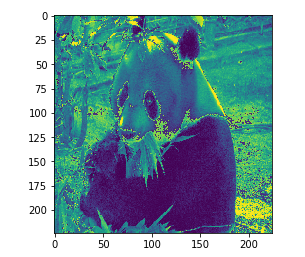# RGB to grayscale, easy

Hi everyone, I was wondering if anyone could explain to me why my code below did not work, I know that RGB conversion to grayscale is (R + G +B/3) so I used PyTorch to extract each channel, then add three of them and divide by 3, but the end result was a distorted image. I viewed my image output using Jupyter notebook. I was successful ultimate importing torch vision and using “transforms functional to gray scale” but still was wondering why my average of the RGB channels didn’t work, I’m assuming I didn’t actually obtain the individual 3 channels seperately or properly average them…

panda = np.array(Image.open(‘panda.jpg’).resize( (224,224)))
panda_tensor = torch.from_numpy(panda)

panda_tensor.size()
print ( panda_tensor.size() )

# Display panda

plt.imshow(panda)

chan_r = panda_tensor[:,:,0].numpy()
chan_g = panda_tensor[:,:,1].numpy()
chan_b = panda_tensor[:,:,2].numpy()

result = (chan_r + chan_g + chan_b/3)

plt.imshow(result)# torch vision grayscale

torchvision.transforms.functional.to_grayscale(Image.open(‘panda.jpg’).resize((224,224)), num_output_channels=1)

The division must be outside the parantheses: `result = (chan_r + chan_g + chan_b)/3`

Furthermore, sometimes different weights are used for converting RGB to gray-scale, like this for example: `0.2989 * R + 0.5870 * G + 0.1140 * B`

3 Likes

This was the original image:Ok, I just the paranthesis the way you said AND divided by 3.0 outside, but it gives me this image:
Whats is going on?Could you check the `dtype` of your tensor?
Usually images are encoded as `uint8` values, which can overflow in such an addition.
Try to cast it to float before the transformation is applied.

1 Like

I know this is an old post but… aside from the possible distortion mentioned, by default plt.imshow will not assume that a rank 2 tensor is a grayscale image and will use the default colormap. If you want to see it as a grayscale image, you have to set cmap=‘gray’ in imshow.